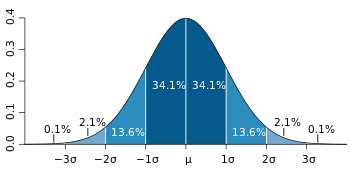# Variance and Standard Deviation Calculator

Share on

This standard deviation calculator calculates the sample standard deviation and variance from a data set. This isn’t your ordinary variance and standard deviation calculator. Type in your numbers and you’ll be given: the variance, the standard deviation, plus you’ll also be able to see your answer step-by-step below.

Watch this short video for instructions, or scroll down to use the calculator:

Data set:

Results

• Variance:
• Standard Deviation:
Explanation

1. First, I added up all of the numbers:
2. I squared the total, and then divided the number of items in the data set
3. I took my set of original numbers from step 1, squared them individually this time, and added them all up:
4. I subtracted the amount in step 2 from the amount in step 3:
5. I subtracted 1 from the number of items in my data set:
6. I divided the number in step 4 by the number in step 5:
7. Finally, I took the square root of the number from step 6 (the Variance),Standard deviations are a unit of measurement; they measure how spread out your data is around the mean. The bulk of data (68%) lies within one standard deviation from the mean.

Like this variance and standard deviation calculator? Share on Facebook with your friends by clicking the Like button at the top of the calculator.

Check out my YouTube channel for hundreds of statistics how to videos!

CITE THIS AS:
Stephanie Glen. "Variance and Standard Deviation Calculator" From StatisticsHowTo.com: Elementary Statistics for the rest of us! https://www.statisticshowto.com/calculators/variance-and-standard-deviation-calculator/
---------------------------------------------------------------------------Need help with a homework or test question? With Chegg Study, you can get step-by-step solutions to your questions from an expert in the field. Your first 30 minutes with a Chegg tutor is free!